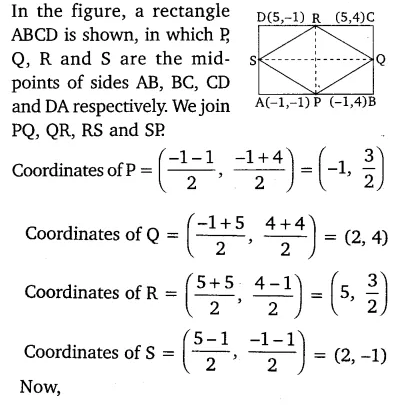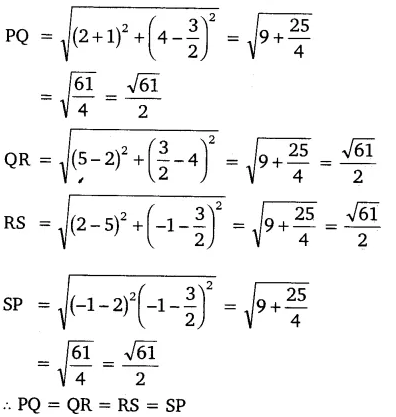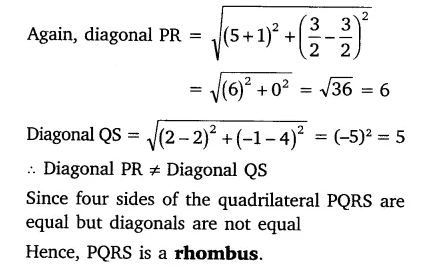# ABCD is a rectangle formed by the points A(-1, -1), B(-1, 4), C(5, 4) and D(5, -1),

ABCD is a rectangle formed by the points A(-1, -1), B(-1, 4), C(5, 4) and D(5, -1), P, Q, R, and S are the mid-points of Ab, BC, CD, and DA respectively. Is the quadrilateral PQRS a square? a rectangle? or a rhombus? Justify your answer.